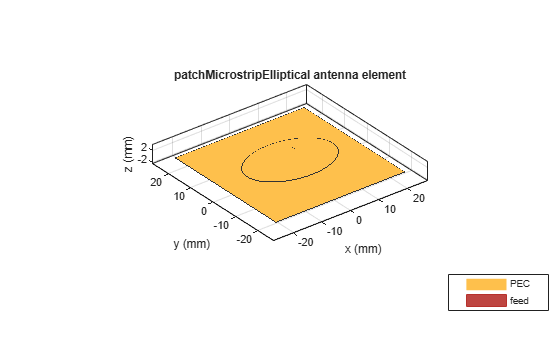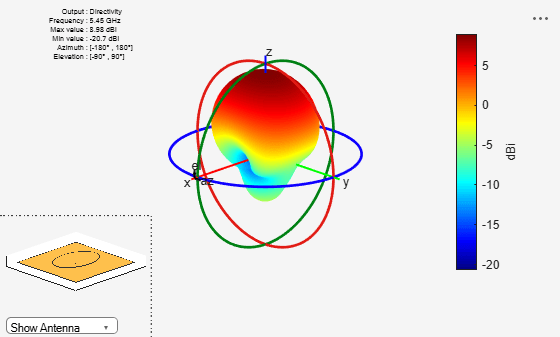# patchMicrostripElliptical

Create elliptical microstrip patch antenna

## Description

The `patchMicrostripElliptical` object creates a probe-fed elliptical microstrip patch antenna. The default patch is centered at the origin. The ellipse is chosen for an operating frequency of around 5.45 GHz. Elliptical microstrip patch antennas are used in high-performance applications such as spacecraft, aircraft, missiles, and satellites. Elliptical microstrip patch antennas with optimum dimensions act as circularly polarized wave radiators.## Creation

### Syntax

``ant = patchMicrostripElliptical``
``ant = patchMicrostripElliptical(Name,Value)``

### Description

example

````ant = patchMicrostripElliptical` creates a probe-fed elliptical microstrip patch antenna operating at 5.45 GHz.```
````ant = patchMicrostripElliptical(Name,Value)` sets properties using one or more name-value pairs. For example, `ant = patchMicrostripElliptical('MajorAxis',0.0878)` creates an elliptical microstrip patch antenna with a major axis of 0.0878 meters. Enclose each property name in quotes.```

## Properties

expand all

Longest diameter of the ellipse along the x-axis, specified as a scalar in meters.

Example: `'MajorAxis',0.0989`

Example: `ant.MajorAxis = 0.0989`

Data Types: `double`

Shortest diameter of the ellipse along the y-axis, specified as a scalar in meters.

Example: `'MinorAxis',0.0898`

Example: `ant.MinorAxis = 0.0898`

Data Types: `double`

Height of patch above the ground plane along the z-axis, specified as a scalar in meters.

Example: `'Height',0.001`

Example: `ant.Height = 0.001`

Data Types: `double`

Type of dielectric material used as a substrate, specified as a dielectric material object. You can choose any material from the `DielectricCatalog` or use your own dielectric material. For more information, see `dielectric`. For more information on dielectric substrate meshing, see Meshing.

Note

The substrate dimensions must be lesser than the ground plane dimensions.

Example: `d = dielectric('FR4'); 'Substrate',d`

Example: `d = dielectric('FR4'); ant.Substrate = d`

Ground plane length along the x-axis, specified as a scalar in meters. Setting `'GroundPlaneLength'` to `Inf`, uses the infinite ground plane technique for antenna analysis.

Example: `'GroundPlaneLength',120e-3`

Example: `ant.GroundPlaneLength = 120e-3`

Data Types: `double`

Ground plane width along the y-axis, specified as a scalar in meters. Setting `'GroundPlaneWidth'` to `Inf`, uses the infinite ground plane technique for antenna analysis.

Example: `'GroundPlaneWidth',120e-3`

Example: `ant.GroundPlaneWidth = 120e-3`

Data Types: `double`

Signed distance of the patch from the origin, specified as a two-element real vector with each element unit in meters. Use this property to adjust the location of the patch relative to the ground plane. Distances are measured along the length and width of the ground plane.

Example: `'PatchCenterOffset',[0.01 0.01]`

Example: ```ant.PatchCenterOffset = [0.01 0.01]```

Data Types: `double`

Signed distance of the feed from the origin, specified as a two-element real vector with each element unit in meters. Use this property to adjust the location of the feed relative to the ground plane and patch.

Example: `'FeedOffset',[0.01 0.01]`

Example: `ant.FeedOffset = [0.01 0.01]`

Data Types: `double`

Type of the metal used as a conductor, specified as a metal material object. You can choose any metal from the `MetalCatalog` or specify a metal of your choice. For more information, see `metal`. For more information on metal conductor meshing, see Meshing.

Example: ```m = metal('Copper'); 'Conductor',m```

Example: ```m = metal('Copper'); ant.Conductor = m```

Lumped elements added to the antenna feed, specified as a `lumpedElement` object. You can add a load anywhere on the surface of the antenna. By default, the load is at the feed. For more information, see `lumpedElement`.

Example: `'Load',lumpedElement`, where `lumpedElement` is load added to the antenna feed.

Example: `ant.Load = lumpedElement('Impedance',75)`

Tilt angle of the antenna, specified as a scalar or vector with each element unit in degrees. For more information, see Rotate Antennas and Arrays.

Example: `Tilt=90`

Example: `Tilt=[90 90]`,`TiltAxis=[0 1 0;0 1 1]` tilts the antenna at 90 degrees about the two axes defined by the vectors.

Note

The `wireStack` antenna object only accepts the dot method to change its properties.

Data Types: `double`

Tilt axis of the antenna, specified as:

• Three-element vector of Cartesian coordinates in meters. In this case, each coordinate in the vector starts at the origin and lies along the specified points on the X-, Y-, and Z-axes.

• Two points in space, each specified as three-element vectors of Cartesian coordinates. In this case, the antenna rotates around the line joining the two points in space.

• A string input describing simple rotations around one of the principal axes, 'X', 'Y', or 'Z'.

For more information, see Rotate Antennas and Arrays.

Example: `TiltAxis=[0 1 0]`

Example: `TiltAxis=[0 0 0;0 1 0]`

Example: `TiltAxis = 'Z'`

Note

The `wireStack` antenna object only accepts the dot method to change its properties.

Data Types: `double`

## Object Functions

 `show` Display antenna, array structures or shapes `axialRatio` Axial ratio of antenna `beamwidth` Beamwidth of antenna `charge` Charge distribution on antenna or array surface `current` Current distribution on antenna or array surface `design` Design prototype antenna or arrays for resonance around specified frequency `efficiency` Radiation efficiency of antenna `EHfields` Electric and magnetic fields of antennas; Embedded electric and magnetic fields of antenna element in arrays `impedance` Input impedance of antenna; scan impedance of array `mesh` Mesh properties of metal, dielectric antenna, or array structure `meshconfig` Change mesh mode of antenna structure `optimize` Optimize antenna or array using SADEA optimizer `pattern` Radiation pattern and phase of antenna or array; Embedded pattern of antenna element in array `patternAzimuth` Azimuth pattern of antenna or array `patternElevation` Elevation pattern of antenna or array `rcs` Calculate and plot radar cross section (RCS) of platform, antenna, or array `returnLoss` Return loss of antenna; scan return loss of array `sparameters` Calculate S-parameter for antenna and antenna array objects `vswr` Voltage standing wave ratio of antenna

## Examples

collapse all

Create and view a default elliptical microstrip patch antenna.

`ant = patchMicrostripElliptical`
```ant = patchMicrostripElliptical with properties: MajorAxis: 0.0300 MinorAxis: 0.0200 Height: 0.0016 Substrate: [1x1 dielectric] GroundPlaneLength: 0.0450 GroundPlaneWidth: 0.0450 PatchCenterOffset: [0 0] FeedOffset: [0.0047 0.0045] Conductor: [1x1 metal] Tilt: 0 TiltAxis: [1 0 0] Load: [1x1 lumpedElement] ```
`show (ant)`Visualize the radiation pattern of the antenna at 5.45 GHz.

`pattern(ant,5.45e9)`## Version History

Introduced in R2020a